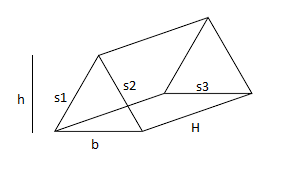# Program to calculate the Surface Area of a Triangular Prism

In mathematics, a triangular prism is a three-dimensional solid shape with two identical ends connected by equal parallel lines, and have 5 faces, 9 edges, and 6 vertices.where “b” is the length of the base, “h” is the height of the triangle, “s1, s2, s3” are the respective length of each side of the triangle, and H is the height of the prism (which is also the length of the rectangle).

Given the base, the height of the triangle, height of prism and the length of each side of triangle base and the task is to calculate the surface area of the triangular prism.

Examples:

Input: b = 3, h = 4, s1 = 3, s2 = 6, s3 = 6, Ht = 8
Output: The area of triangular prism is 132.000000

Input: b = 2, h = 3, s1 = 4, s2 = 5, s3 = 6, Ht = 8
Output: The area of triangular prism is 126.000000

Formula for calculating the surface area:
As stated above, the prism contains two triangles of the area (1/2)*(b)*(h) and three rectangles of the area H*s1, H*s2 and H*s3.
Now after adding all the terms we get the total surface area:

`SA = b * h + (s1 + s2 + s3 ) * H`

## C++

 `// C++ Program to calculate the ` `// Surface area of a triangular prism ` `#include ` `using` `namespace` `std; ` ` `  `// Function for calculating the area ` `void` `Calculate_area() ` `{ ` `    ``// Initialization ` `    ``float` `b = 3, h = 4, s1 = 3, s2 = 6; ` `    ``float` `s3 = 6, Ht = 8, SA; ` ` `  `    ``// Formula for calculating the area ` `    ``SA = b * h + (s1 + s2 + s3) * Ht; ` ` `  `    ``// Displaying the area ` `    ``cout << ``"The area of triangular prism is : "` `<< SA; ` `} ` ` `  `// Driver code ` `int` `main() ` `{ ` `    ``// Function calling ` `    ``Calculate_area(); ` ` `  `    ``return` `0; ` `} `

## C

 `// C Program to calculate the ` `// Surface area of a triangular prism ` `#include ` ` `  `// Function for calculating the area ` `void` `Calculate_area() ` `{ ` `    ``// Initialization ` `    ``float` `b = 3, h = 4, s1 = 3, s2 = 6; ` `    ``float` `s3 = 6, Ht = 8, SA; ` ` `  `    ``// Formula for calculating the area ` `    ``SA = b * h + (s1 + s2 + s3) * Ht; ` ` `  `    ``// Displaying the output ` `    ``printf``(``"The area of triangular prism is : %f"``, SA); ` `} ` ` `  `// Driver code ` `int` `main() ` `{ ` `    ``// Function calling ` `    ``Calculate_area(); ` ` `  `    ``return` `0; ` `} `

## Java

 `// Java Program to calculate the ` `// Surface area of a triangular prism ` ` `  `import` `java.util.Scanner; ` `public` `class` `Prism { ` ` `  `    ``public` `static` `void` `Calculate_area() ` `    ``{ ` `        ``// Initialization ` `        ``double` `b = ``3``, h = ``4``, s1 = ``3``, s2 = ``6``; ` `        ``double` `s3 = ``6``, Ht = ``8``, SA; ` ` `  `        ``// Formula for calculating the area ` `        ``SA = b * h + (s1 + s2 + s3) * Ht; ` ` `  `        ``// Displaying the area ` `        ``System.out.printf(``"The area of triangular prism is : %f"``, SA); ` `    ``} ` `    ``public` `static` `void` `main(String[] args) ` `    ``{ ` `        ``Calculate_area(); ` `    ``} ` `} ` `// This code is contributed by Nishant Tanwar `

## Python3

 `# Python3 Program to calculate the ` `# Surface area of a triangular prism ` ` `  `# Function for calculating the area ` `def` `Calculate_area(): ` `     `  `    ``# Initialization ` `    ``b ``=` `3` `    ``h ``=` `4` `    ``s1 ``=` `3` `    ``s2 ``=` `6` `    ``s3 ``=` `6` `    ``Ht ``=` `8` ` `  `    ``# Formula for calculating the area ` `    ``SA ``=` `b ``*` `h ``+` `(s1 ``+` `s2 ``+` `s3) ``*` `Ht ` ` `  `    ``# Displaying the area ` `    ``print` `(``"The area of triangular prism is :"``,SA) ` ` `  `# Driver code ` `if` `__name__ ``=``=` `'__main__'``: ` `    ``# Function calling ` `    ``Calculate_area() ` ` `  `# This code is contributed by  ` `# Surendra_Gangwar `

## C#

 `// C# Program to calculate the ` `// Surface area of a triangular prism ` `using` `System; ` `public` `class` `Prism { ` `  `  `    ``static` `void` `Calculate_area() ` `    ``{ ` `        ``// Initialization ` `        ``double` `b = 3, h = 4, s1 = 3, s2 = 6; ` `        ``double` `s3 = 6, Ht = 8, SA; ` `  `  `        ``// Formula for calculating the area ` `        ``SA = b * h + (s1 + s2 + s3) * Ht; ` `  `  `        ``// Displaying the area ` `        ``Console.WriteLine(``"The area of triangular prism is : "` `+ SA); ` `    ``} ` `    ``static` `public` `void` `Main(String[] args) ` `    ``{ ` `        ``Calculate_area(); ` `    ``} ` `} `

## PHP

 ` `

Output:

```The area of triangular prism is : 132.000000
```

Don’t stop now and take your learning to the next level. Learn all the important concepts of Data Structures and Algorithms with the help of the most trusted course: DSA Self Paced. Become industry ready at a student-friendly price.

My Personal Notes arrow_drop_upCheck out this Author's contributed articles.

If you like GeeksforGeeks and would like to contribute, you can also write an article using contribute.geeksforgeeks.org or mail your article to contribute@geeksforgeeks.org. See your article appearing on the GeeksforGeeks main page and help other Geeks.

Please Improve this article if you find anything incorrect by clicking on the "Improve Article" button below.